# How to Auto Convert KB, MB, GB, PG, TB in Excel

This post will guide you how to convert value in KB format to MB, GB, PB and TB automatically in Microsoft Excel. How do I convert size in bytes to KB, MB, GB, TB and PB in Excel 2013/2016.

For example, assume that you have a list of data  which unit is bytes, and you wish to convert the unit of these values to KB, MB, GB, TB. You should know that the unit conversion rate is 1024 between each adjacent unit. So you can create a formula similar as the below:

=B1/1024

If you want to convert the unit from KB to MB, and you can type the following formula into a blank cell, then press Enter key.

=B2/1024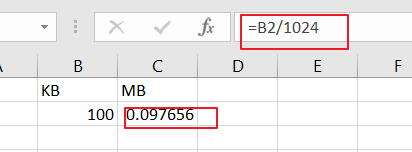If you want to convert the unit from byte to MB, and you can use the following formula:

=B1/1024/1024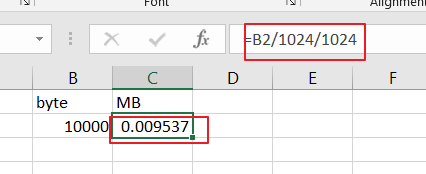Or you can also use another formula based on the CONVERT function to convert a number from one measurement system to another.

The syntax of the Convert function is as below:

=CONVERT (number, from_unit, to_unit)

Where,

Number – cell values that you want to convert its unit.

From_unit – the source unit

To_unit – the target unit

The prefixes of from/to unit should be Kibyte, Mibyte, Gibyte and Tibyte.

### Convert KB to MB

To convert size in KB to MB, and you can use the following formula:

=CONVERT(B1,”kibyte”,”Mibyte”)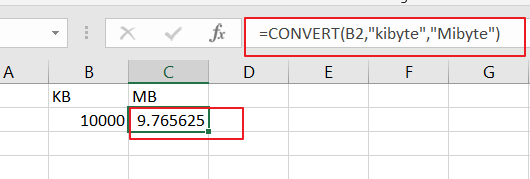### Convert MB to GB

If you wish to convert the unit from MB to GB, and you can use the following convert formula:

=CONVERT(B2,”Mibyte”,”Gibyte”)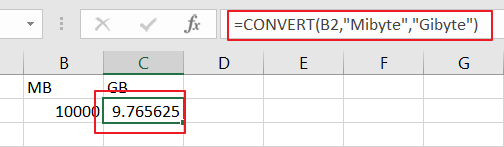### Convert GB to TB

If the unit of your original data is MB, and you wish to convert it as GB, just use the below Convert formula:

=CONVERT(D2,”Gibyte”,”Tibyte”)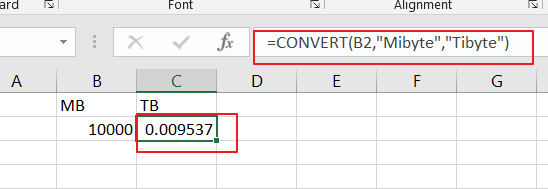Sidebar